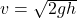## Two balls are dropped (with zero initial speed) from a height h. show answer No Attempt If ball A has twice the mass of ball B, what can be

Question

Two balls are dropped (with zero initial speed) from a height h. show answer No Attempt If ball A has twice the mass of ball B, what can be said about the momentum of each just before they hit the ground? Ignore air resistance.

in progress 0
2 weeks 2021-08-29T03:05:10+00:00 1 Answers 0 views 0

Explanation:

Let the mass of ball B is m so that the mass of ball A is 2m.

initial velocity of both the balls = 0

Let both the balls falls from the height h.

Use third equation of motion,

v² = u² + 2gh

so, the velocity with which the balls hit the ground isSo, both the balls hit the ground with the same speed.

momentum, p = mass x velocity

As the velocity is same but the mass of ball A is 2 times the mass of ball B, so the momentum of ball A is twice the momentum of ball A.